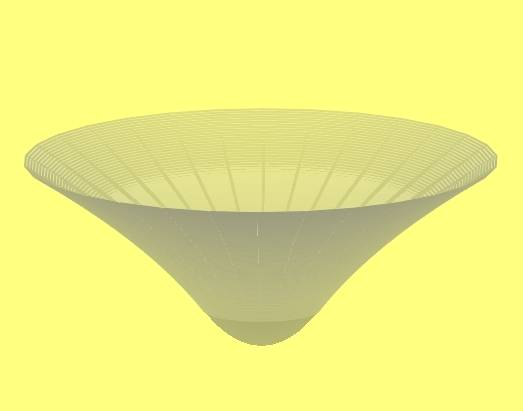# Is Flamm's paraboloid a paraboloid?

• I
• pchu

#### pchu

The shape of the Einstein-Rosen bridge is often visualized/modelized with the Flamm's paraboloid, and many other references have also stated clearly that it's a "surface of revolution of a parabola".
But as far as I can see, when we rotate the parabola w^2 = 8M(r-2M) (in natural units c=G=1) around the w axis (i.e r=0), we get no paraboloid.
The rotation process replaces r coordinate by sqrt(x^2+y^2), so that the resulting surface equation becomes
(w^2/8M+2M)^2 = x^2+y^2
This is even not a quadric surface, let alone a paraboloid (which looks like a bowl).
Only the rotation around its symmetric axis (i.e r axis) gives a paraboloid of revolution!
Instead, the shape of the Einstein-Rosen bridge rather looks like a hyperboloid of 1 sheet (although not rigorously).

What's annoying me is that I cannot find anyone / any article on the Internet highlighting the fact that the Flamm's paraboloid is NOT a paraboloid, am I wrong??

This is even not a quadric surface, let alone a paraboloid (which looks like a bowl).
Maybe you rotate it around the wrong axis?

Maybe you rotate it around the wrong axis?
But if we rotate it around the correct'' axis to get the real paraboloid of revolution: https://en.wikipedia.org/wiki/Paraboloid
Now it looks nothing like the Flamm's paraboloid'' (or, Einstein-Rosen bridge)...

Last edited:
From the exterior a black hole pinches a hole in spacetime so it is cut of at r=2GM/c², but when you look at the interior Schwarzschild solution you get the paraboloid in the complex plane:From the exterior a black hole pinches a hole in spacetime so it is cut of at r=2GM/c², but when you look at the interior Schwarzschild solution you get the paraboloid in the complex plane:This is wrong.

Spacetime is not cut at r=2GM/c² and the interior part is not parabolic but spherical.

The interior Schwarzschild solution describes what happens in a spherically symmetric incompressible fluid with zero pressure on the surface. Outside you will have the regular Schwartzschild vacuum solution and the transition between the two does not occur at the Schwarzschild radius, but wherever the surface of the fluid is.

a black hole pinches a hole in spacetime so it is cut of at r=2GM/c²

No, this is not correct. What is correct for a black hole is that the spacelike surfaces of constant Schwarzschild coordinate time ##t## in the exterior of Schwarzschild spacetime (i.e., above the horizon), whose spatial geometry is described by the Flamm paraboloid, do not extend below the horizon. This is usually reflected by cutting off the paraboloid at ##r = 2M##. But strictly speaking, the maximally extended spacetime has another exterior region, so the full spacelike surface is actually two paraboloids joined at the ##r = 2M## surface. There is no "hole in spacetime" at ##r = 2M##.

•vanhees71
From the exterior a black hole pinches a hole in spacetime so it is cut of at r=2GM/c², but when you look at the interior Schwarzschild solution you get the paraboloid in the complex plane:No, that's the picture for spherical incompressible fluid in vacuum, which has nothing to do with the Einstein-Rosen bridge model.

No, this is not correct. What is correct for a black hole is that the spacelike surfaces of constant Schwarzschild coordinate time ##t## in the exterior of Schwarzschild spacetime (i.e., above the horizon), whose spatial geometry is described by the Flamm paraboloid, do not extend below the horizon. This is usually reflected by cutting off the paraboloid at ##r = 2M##. But strictly speaking, the maximally extended spacetime has another exterior region, so the full spacelike surface is actually two paraboloids joined at the ##r = 2M## surface. There is no "hole in spacetime" at ##r = 2M##.
But then the Flamm's paraboloid looks nothing like a well-defined paraboloid!?
Can we just rotate a parabola around an arbitrary line and call that a paraboloid!?

Can we just rotate a parabola around an arbitrary line and call that a paraboloid!?

It depends on how fussy you want to be about terminology. The way the Flamm paraboloid is generated is perfectly clear, and the math describing it is unambiguous. Whether you think it deserves the name "paraboloid" is a question of terminology, not math or physics.

Can we just rotate a parabola around an arbitrary line and call that a paraboloid!?
It's called "Flamm's paraboloid" not just "paraboloid".

It depends on how fussy you want to be about terminology. The way the Flamm paraboloid is generated is perfectly clear, and the math describing it is unambiguous. Whether you think it deserves the name "paraboloid" is a question of terminology, not math or physics.
Alright, then we do agree that this "Flamm's paraboloid" is NOT what mathematicians defined as "paraboloid", that whenever we mention it, we should call it "Flamm's paraboloid" as a whole, right?
I think that it's like we don't rotate an ellipse around an arbitrary inclined axis and call that an ellipsoid...

we do agree that this "Flamm's paraboloid" is NOT what mathematicians defined as "paraboloid"

It would appear that it isn't according to the definition you referenced, no.

whenever we mention it, we should call it "Flamm's paraboloid" as a whole

That's how I've always seen it referred to in GR discussions, yes.

That's how I've always seen it referred to in GR discussions, yes.
Lucky for you, cause I've seen people calling it paraboloid surface or something in several literatures.
In another book, the author even explains that the paraboloid is a parabola rotating around its axis of symmetry (which is generally correct but not in this case), which made me feel WTF for a second... (ok maybe that's a typo)

[Moderator's note: a subthread on the spatial geometry of constant Schwarzschild time hypersurfaces in the maximally extended spacetime has been spun off to a different thread.]